This book is archived and will be removed July 6, 2022. Please use the updated version.

Magnetic Forces and Fields

# 70 Magnetic Fields and Lines

### Learning Objectives

By the end of this section, you will be able to:

• Define the magnetic field based on a moving charge experiencing a force
• Apply the right-hand rule to determine the direction of a magnetic force based on the motion of a charge in a magnetic field
• Sketch magnetic field lines to understand which way the magnetic field points and how strong it is in a region of space

We have outlined the properties of magnets, described how they behave, and listed some of the applications of magnetic properties. Even though there are no such things as isolated magnetic charges, we can still define the attraction and repulsion of magnets as based on a field. In this section, we define the magnetic field, determine its direction based on the right-hand rule, and discuss how to draw magnetic field lines.

### Defining the Magnetic Field

A magnetic field is defined by the force that a charged particle experiences moving in this field, after we account for the gravitational and any additional electric forces possible on the charge. The magnitude of this force is proportional to the amount of charge q, the speed of the charged particle v, and the magnitude of the applied magnetic field. The direction of this force is perpendicular to both the direction of the moving charged particle and the direction of the applied magnetic field. Based on these observations, we define the magnetic field strength B based on the magnetic force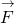on a charge q moving at velocity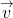as the cross product of the velocity and magnetic field, that is,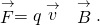In fact, this is how we define the magnetic field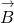—in terms of the force on a charged particle moving in a magnetic field. The magnitude of the force is determined from the definition of the cross product as it relates to the magnitudes of each of the vectors. In other words, the magnitude of the force satisfies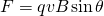where θ is the angle between the velocity and the magnetic field.

The SI unit for magnetic field strength B is called the tesla (T) after the eccentric but brilliant inventor Nikola Tesla (1856–1943), where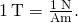A smaller unit, called the gauss (G), where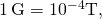is sometimes used. The strongest permanent magnets have fields near 2 T; superconducting electromagnets may attain 10 T or more. Earth’s magnetic field on its surface is only about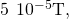or 0.5 G.

Problem-Solving Strategy: Direction of the Magnetic Field by the Right-Hand Rule

The direction of the magnetic forceis perpendicular to the plane formed byand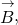as determined by the right-hand rule-1 (or RHR-1), which is illustrated in (Figure).

1. Orient your right hand so that your fingers curl in the plane defined by the velocity and magnetic field vectors.
2. Using your right hand, sweep from the velocity toward the magnetic field with your fingers through the smallest angle possible.
3. The magnetic force is directed where your thumb is pointing.
4. If the charge was negative, reverse the direction found by these steps.
Magnetic fields exert forces on moving charges. The direction of the magnetic force on a moving charge is perpendicular to the plane formed byandand follows the right-hand rule-1 (RHR-1) as shown. The magnitude of the force is proportional to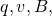and the sine of the angle betweenand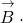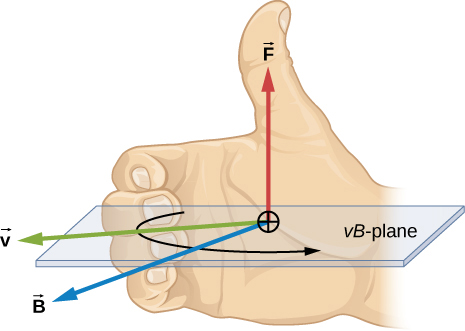Visit this website for additional practice with the direction of magnetic fields.

There is no magnetic force on static charges. However, there is a magnetic force on charges moving at an angle to a magnetic field. When charges are stationary, their electric fields do not affect magnets. However, when charges move, they produce magnetic fields that exert forces on other magnets. When there is relative motion, a connection between electric and magnetic forces emerges—each affects the other.

An Alpha-Particle Moving in a Magnetic Field An alpha-particle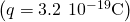moves through a uniform magnetic field whose magnitude is 1.5 T. The field is directly parallel to the positive z-axis of the rectangular coordinate system of (Figure). What is the magnetic force on the alpha-particle when it is moving (a) in the positive x-direction with a speed of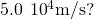(b) in the negative y-direction with a speed of(c) in the positive z-direction with a speed of(d) with a velocity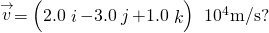The magnetic forces on an alpha-particle moving in a uniform magnetic field. The field is the same in each drawing, but the velocity is different.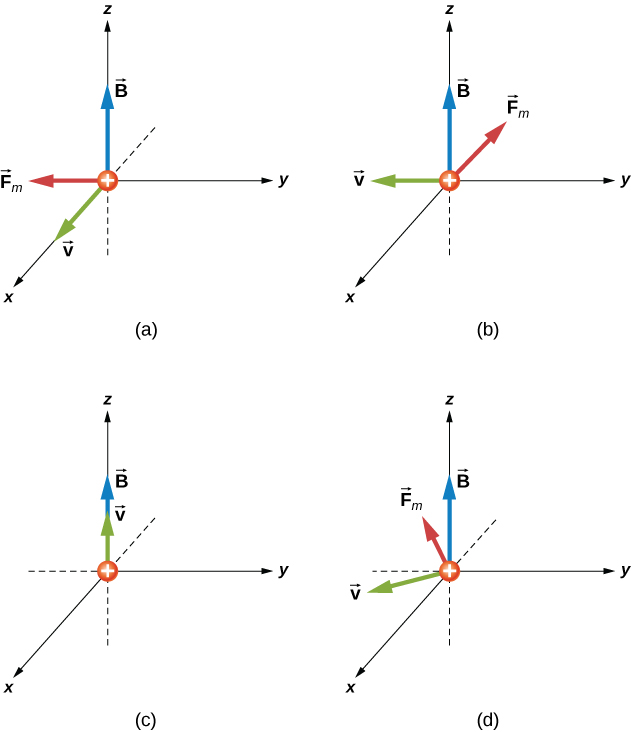Strategy We are given the charge, its velocity, and the magnetic field strength and direction. We can thus use the equation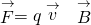orto calculate the force. The direction of the force is determined by RHR-1.

Solution

1. First, to determine the direction, start with your fingers pointing in the positive x-direction. Sweep your fingers upward in the direction of magnetic field. Your thumb should point in the negative y-direction. This should match the mathematical answer. To calculate the force, we use the given charge, velocity, and magnetic field and the definition of the magnetic force in cross-product form to calculate: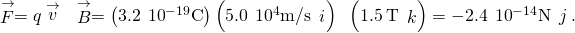2. First, to determine the directionality, start with your fingers pointing in the negative y-direction. Sweep your fingers upward in the direction of magnetic field as in the previous problem. Your thumb should be open in the negative x-direction. This should match the mathematical answer. To calculate the force, we use the given charge, velocity, and magnetic field and the definition of the magnetic force in cross-product form to calculate: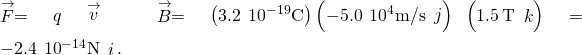An alternative approach is to use (Figure) to find the magnitude of the force. This applies for both parts (a) and (b). Since the velocity is perpendicular to the magnetic field, the angle between them is 90 degrees. Therefore, the magnitude of the force is: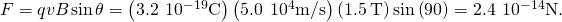3. Since the velocity and magnetic field are parallel to each other, there is no orientation of your hand that will result in a force direction. Therefore, the force on this moving charge is zero. This is confirmed by the cross product. When you cross two vectors pointing in the same direction, the result is equal to zero.
4. First, to determine the direction, your fingers could point in any orientation; however, you must sweep your fingers upward in the direction of the magnetic field. As you rotate your hand, notice that the thumb can point in any x– or y-direction possible, but not in the z-direction. This should match the mathematical answer. To calculate the force, we use the given charge, velocity, and magnetic field and the definition of the magnetic force in cross-product form to calculate: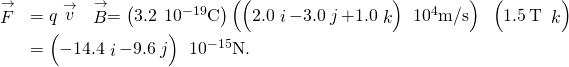This solution can be rewritten in terms of a magnitude and angle in the xy-plane: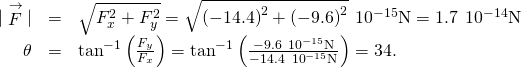The magnitude of the force can also be calculated using (Figure). The velocity in this question, however, has three components. The z-component of the velocity can be neglected, because it is parallel to the magnetic field and therefore generates no force. The magnitude of the velocity is calculated from the x– and y-components. The angle between the velocity in the xy-plane and the magnetic field in the z-plane is 90 degrees. Therefore, the force is calculated to be: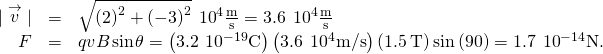This is the same magnitude of force calculated by unit vectors.

Significance The cross product in this formula results in a third vector that must be perpendicular to the other two. Other physical quantities, such as angular momentum, also have three vectors that are related by the cross product. Note that typical force values in magnetic force problems are much larger than the gravitational force. Therefore, for an isolated charge, the magnetic force is the dominant force governing the charge’s motion.

Check Your Understanding Repeat the previous problem with the magnetic field in the x-direction rather than in the z-direction. Check your answers with RHR-1.

a. 0 N; b.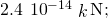c.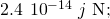d.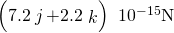### Representing Magnetic Fields

The representation of magnetic fields by magnetic field lines is very useful in visualizing the strength and direction of the magnetic field. As shown in (Figure), each of these lines forms a closed loop, even if not shown by the constraints of the space available for the figure. The field lines emerge from the north pole (N), loop around to the south pole (S), and continue through the bar magnet back to the north pole.

Magnetic field lines have several hard-and-fast rules:

1. The direction of the magnetic field is tangent to the field line at any point in space. A small compass will point in the direction of the field line.
2. The strength of the field is proportional to the closeness of the lines. It is exactly proportional to the number of lines per unit area perpendicular to the lines (called the areal density).
3. Magnetic field lines can never cross, meaning that the field is unique at any point in space.
4. Magnetic field lines are continuous, forming closed loops without a beginning or end. They are directed from the north pole to the south pole.

The last property is related to the fact that the north and south poles cannot be separated. It is a distinct difference from electric field lines, which generally begin on positive charges and end on negative charges or at infinity. If isolated magnetic charges (referred to as magnetic monopoles) existed, then magnetic field lines would begin and end on them.

Magnetic field lines are defined to have the direction in which a small compass points when placed at a location in the field. The strength of the field is proportional to the closeness (or density) of the lines. If the interior of the magnet could be probed, the field lines would be found to form continuous, closed loops. To fit in a reasonable space, some of these drawings may not show the closing of the loops; however, if enough space were provided, the loops would be closed.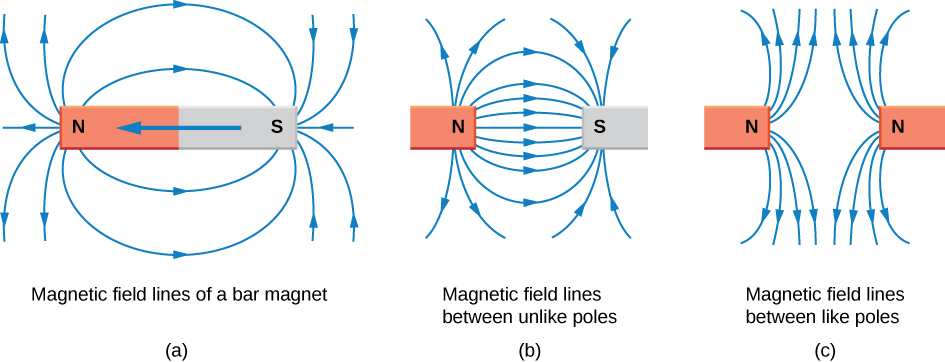### Summary

• Charges moving across a magnetic field experience a force determined byThe force is perpendicular to the plane formed byand• The direction of the force on a moving charge is given by the right hand rule 1 (RHR-1): Sweep your fingers in a velocity, magnetic field plane. Start by pointing them in the direction of velocity and sweep towards the magnetic field. Your thumb points in the direction of the magnetic force for positive charges.
• Magnetic fields can be pictorially represented by magnetic field lines, which have the following properties:
1. The field is tangent to the magnetic field line.
2. Field strength is proportional to the line density.
3. Field lines cannot cross.
4. Field lines form continuous, closed loops.
• Magnetic poles always occur in pairs of north and south—it is not possible to isolate north and south poles.

### Conceptual Questions

Discuss the similarities and differences between the electrical force on a charge and the magnetic force on a charge.

Both are field dependent. Electrical force is dependent on charge, whereas magnetic force is dependent on current or rate of charge flow.

(a) Is it possible for the magnetic force on a charge moving in a magnetic field to be zero? (b) Is it possible for the electric force on a charge moving in an electric field to be zero? (c) Is it possible for the resultant of the electric and magnetic forces on a charge moving simultaneously through both fields to be zero?

### Problems

What is the direction of the magnetic force on a positive charge that moves as shown in each of the six cases?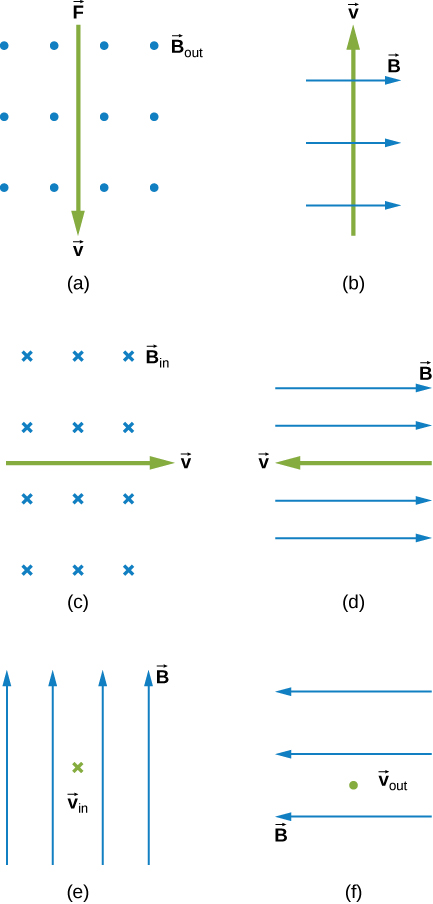a. left; b. into the page; c. up the page; d. no force; e. right; f. down

Repeat previous exercise for a negative charge.

What is the direction of the velocity of a negative charge that experiences the magnetic force shown in each of the three cases, assuming it moves perpendicular to B?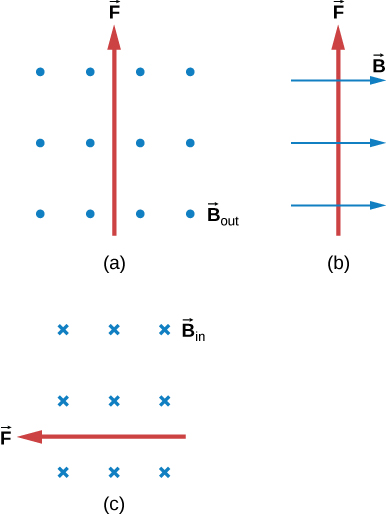a. right; b. into the page; c. down

Repeat previous exercise for a positive charge.

What is the direction of the magnetic field that produces the magnetic force on a positive charge as shown in each of the three cases, assumingis perpendicular to?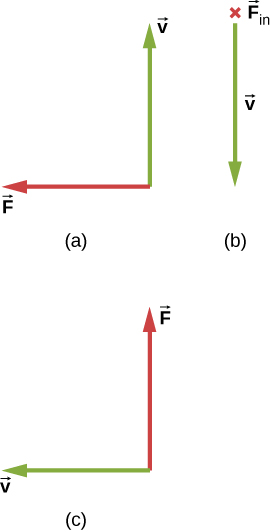a. into the page; b. left; c. out of the page

Repeat previous exercise for a negative charge.

(a) Aircraft sometimes acquire small static charges. Suppose a supersonic jet has a 0.500-μC charge and flies due west at a speed of 660. m/s over Earth’s south magnetic pole, where the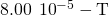magnetic field points straight down into the ground. What are the direction and the magnitude of the magnetic force on the plane? (b) Discuss whether the value obtained in part (a) implies this is a significant or negligible effect.

a.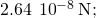north b. The force is very small, so this implies that the effect of static charges on airplanes is negligible.

(a) A cosmic ray proton moving toward Earth at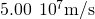experiences a magnetic force of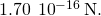What is the strength of the magnetic field if there is a 45º angle between it and the proton’s velocity? (b) Is the value obtained in part a. consistent with the known strength of Earth’s magnetic field on its surface? Discuss.

An electron moving at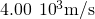in a 1.25-T magnetic field experiences a magnetic force of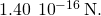What angle does the velocity of the electron make with the magnetic field? There are two answers.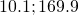(a) A physicist performing a sensitive measurement wants to limit the magnetic force on a moving charge in her equipment to less than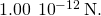What is the greatest the charge can be if it moves at a maximum speed of 30.0 m/s in Earth’s field? (b) Discuss whether it would be difficult to limit the charge to less than the value found in (a) by comparing it with typical static electricity and noting that static is often absent.

### Glossary

gauss
G, unit of the magnetic field strength;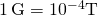magnetic field lines
continuous curves that show the direction of a magnetic field; these lines point in the same direction as a compass points, toward the magnetic south pole of a bar magnet
magnetic force
force applied to a charged particle moving through a magnetic field
right-hand rule-1
using your right hand to determine the direction of either the magnetic force, velocity of a charged particle, or magnetic field
tesla
SI unit for magnetic field: 1 T = 1 N/A-m